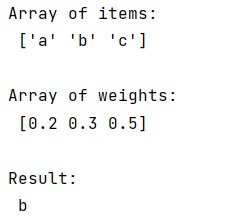# Weighted choice short and simple

Learn, how to choose some items from a list according to another list values?
Submitted by Pranit Sharma, on February 16, 2023

NumPy is an abbreviated form of Numerical Python. It is used for different types of scientific operations in python. Numpy is a vast library in python which is used for almost every kind of scientific or mathematical operation. It is itself an array which is a collection of various methods and functions for processing the arrays.

Suppose that we are given a list of items and we need to choose some items from this list based on another list of weights that is given to us.

We need to select some items from the list randomly based on the weights given in another list.

For this purpose, we will use numpy.random.choice() which generates a random sample from a given 1-D array.

It also takes an argument called p which is also a 1D array. These are the probabilities associated with each entry in the given 1D array. If not given the sample assumes a uniform distribution over all entries in a 1D array.

We will pass the array of items in this method and also pass the array of weights in place of p.

Let us understand with the help of an example,

## Python code for weighted choice short and simple

```# Import numpy
import numpy as np

# Creating two numpy arrays
arr = np.array(['a','b','c'])
weg = np.array([0.2, 0.3, 0.5])

# Display original arrays
print("Array of items:\n",arr,"\n")
print("Array of weights:\n",weg,"\n")

# Selcting random items based on weights
res = np.random.choice(arr,p=weg)

# Display Result
print("Result:\n",res)
```

Output: